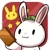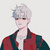实现整型除法四舍五入

【福利】CTO直接拍板说好用的SDK全在这里了！0元配齐，全部免费！>>>1x / y，判断与x相近的上下两个y的倍数，谁跟x更近

int round_div(int x,int y){
int result=x/y;
int remainder=x%y;
if (remainder==0){
return result;
}
int lower_bound=x-remainder;
int upper_bound=lower_bound+y;
return upper_bound-x > x-lower_bound?
result:
upper_bound/y;
}

c

0
c

round是对浮点型用的啊
0

010.5/2=5.25    5.25+0.5=5.75 -> 5

11.4/2=5.7      5.7+0.5=6.2 -> 6